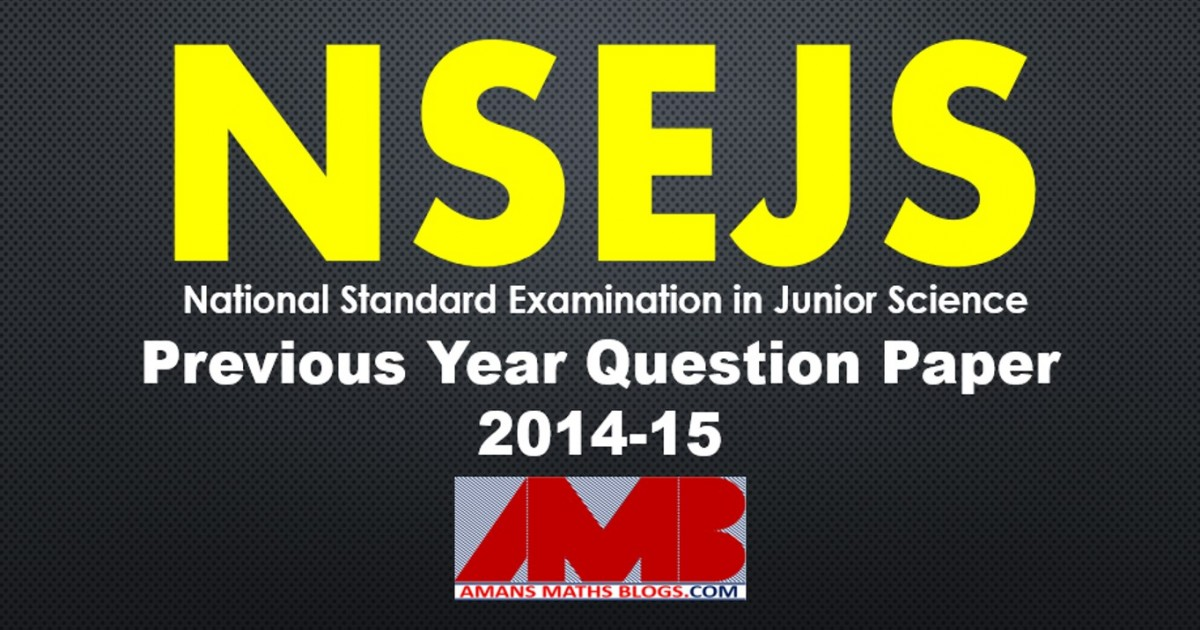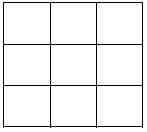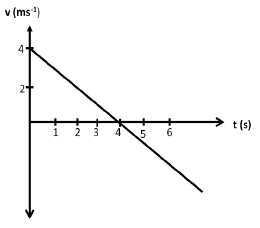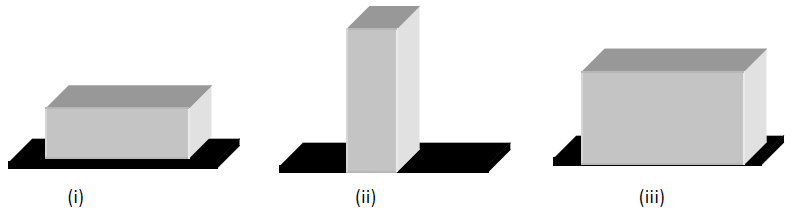Friday, October 22, 2021

# NSEJS PREVIOUS PAPERS 2014-2015# NSEJS PREVIOUS PAPERS 2014-2015

In this post, you will get NSEJS Previous Papers 2014-2015. NSEJS is the first phase of IJSO, it is organized by the IAPT with HBCSE. The full form of NSEJS is National Standard Examination in Junior Science.

NSEJS
Previous Year Question Papers
2008-092009-102010-112011-122012-13
2013-142014-152015-162016-172017-18
2018-192019-20

PART A : Only ONE out of four options is correct

Read More : 129 Maths Short Tricks

NSEJS Previous Paper 2014-2015 Ques No 1:

Three identical vessels carrying equal amount of water are placed in three lifts. Lift A is accelerating upwards, lift B is accelerating downwards while lift C is moving up with constant velocity. The pressure at a depth h from free surface in the three vessel is measured as pA , pB and pc then which of the following is true

Options:

A. pA = pC > pB

B. pA > pC > pB

C. pA > pC = pB

D. pA = pC = pB

NSEJS Previous Papers 2014-2015 Ques No 2:

In the reaction 2KClO3 → 2KCl + 3O2. What is the volume of oxygen released under NTP conditions when 36.75g of KClO3 is heated?

Options:

A. 3.6 litres

B. 7.2 litres

C. 10 litres

D. 1.8 litres

## NSEJS Maths Questions Bank EBook

Get NSEJS 2008 to 2018 Maths Questions Bank with Solutions. To get this combo ebook, click below RED button.NSEJS Previous Papers 2014-2015 Ques No 3:

Figure shows a square grid of order 3, which of the following is correct formula for the total number of squares in a similar grid of order n.Options:

A. n(n + 1)/2

B. n(n + 1)(2n + 1)/6

C. n2(n + 1)2/4

D. n(n + 1)(n + 2)/6

NSEJS Previous Papers 2014-2015 Ques No 4:

If the distance between genes – W. X. Y, and Z on a chromosome are as follows: from W-Y is
18 units, W-X is 26 units, W-Z is 40 units, X-Y is 8 units and X-Z is 14 units, the sequence of W, X, Y, Z genes on the chromosome would be:

Options:

A. W, X, Y, Z.

B. X, Y, W, Z.

C. Y, W, X, Z.

D. W, Y, X, Z.

NSEJS Previous Papers 2014-2015 Ques No 5:

In a plant, 30 megaspore mother cells are generated. If all the ovules are fertilised, how many seeds are expected to be formed

Options:

A. 30

B. 60

C. 90

D. 120

NSEJS Previous Papers 2014-2015 Ques No 6:

A water filter advertisement claims to provide 8 litres of water per hour. How much time does it take to fill four bottles of 1.5 litres each

Options:

A. 2 hr

B. 1 hr

C. 45 min

D. 30 min

NSEJS Previous Papers 2014-2015 Ques No 7:

Which among the following salts will not change the pH of water on addition?

Options:

A. Sodium chloride

B. Sodium cyanide

C. Sodium bicarbonate

D. Sodium carbonate

NSEJS Previous Papers 2014-2015 Ques No 8:

A particle starting from rest is moving with uniform acceleration in a straight line. The percentage increase of the displacement of the particle in 9th second compared to that in the immediate previous second is about

Options:

A. 8.3%

B. 13.3%

C. 20.6%

D. 24.5%

NSEJS Previous Papers 2014-2015 Ques No 9:

An inflated balloon with a heavy rock tied to it submerges in water. As the balloon sinks deeper and deeper, the buoyant force acting on it

Options:

A. increases

B. decreases

C. remains nearly unchanged

D. Initially increases and then decreases

NSEJS Previous Papers 2014-2015 Ques No 10:

For a first order reaction, the ratio of the times taken for completion of 99.9% and 50% of the reaction is

Options:

A. 8

B. 9

C. 10

D. 12

NSEJS Previous Papers 2014-2015 Ques No 11:

If set of marbles, of radius 5 cm, is poured into a cube of side 1 m. The maximum number of marbles that can be filled into the box are

Options:

A. 1000

B. 2000

C. 1500

D. 3000

NSEJS Previous Papers 2014-2015 Ques No 12:

Most of the insects have egg, larva, pupa and adult stages in the life cycle. This is primarily due to :

Options:

B. terrestrial habitat they have adapted to

C. eggs storing little reserved food.

D. flying mode of locomotion majority have

NSEJS Previous Papers 2014-2015 Ques No 13:

Which of the following has been proved to contribute to the transport of water in vascular plants?

(i) Positive root pressure

(ii) Hyrophilic cell walls

(iii) Capillarity

(iv) Transpirational pull

(v) Cohesion between water molecules

Options:

A. i, ii, iii, iv and v

B. only I, iii and v

C. only ii, iv and v

D. only I, ii, iv and v

NSEJS Previous Papers 2014-2015 Ques No 14:

A round table cover has six equal designs as shown in the adjacent figure. If the radius of the cover is 4 cm, then cost of making the designs at the rate of Rs 10.00 per cm2 (round off your answer to a nearest rupee) isOptions:

A. Rs. 85

B. Rs. 86

C. Rs. 87

D. Rs. 90

NSEJS Previous Papers 2014-2015 Ques No 15:

Which of the following series of elements have nearly the same atomic radii?

Options:

A. F, Cl, Br, I

B. Na, K, Rb, Cs

C. Li, Be, B, C

D. Fe, Co, Ni, Cu

NSEJS Previous Papers 2014-2015 Ques No 16:

A particle is moving along a straight line. Its velocity time graph is as shown in the adjacent figure. Then Match the following:Options:

A. (i) is (p); (ii) is (q) and (iii) is (r)

B. (i) is (q); (ii) is (r) and (iii) is (p)

C. (i) is (r); (ii) is (r) and (iii) is (p)

D. (i) is (q); (ii) is (p) and (iii) is (r)

NSEJS Previous Papers 2014-2015 Ques No 17:

A rectangular parallelepiped with sides a, b and c in the ratio 3:2:1 is kept on a uniformly rough horizontal surface as shown in the figures below. The value of limiting friction isOptions:

A. Minimum in (i)

B. Minimum in (ii)

C. Minimum in (iii)

D. Same in all cases

NSEJS Previous Paper 2014-2015 Ques No 18:

Which of the following has the maximum number of unpaired electrons?

Options:

A. Ti 3+

B. V 3+

C. Fe3+

D. Fe2+

NSEJS Previou Paper 2014-2015 Ques No 19:

The houses of a row are numbered consecutively from 1 to 49. Find the value of x such that the sum of the numbers of houses preceding the house numbered x is equal to the sum of the numbers of the houses following it.

Options:

A. 25

B. 37

C. 35

D. None of these

NSEJS Previou Papers 2014-2015 Ques No 20:

Urea is the principle excretary waste in larval as well as adult phases of:

Options:

A. Cockroach

B. Frog

C. Crab

D. Starfish

NSEJS
Previous Year Question Papers
2008-092009-102010-112011-122012-13
2013-142014-152015-162016-172017-18
2018-192019-20

## NSEJS Maths Questions Bank EBook

Get NSEJS 2008 to 2018 Maths Questions Bank with Solutions. To get this combo ebook, click below RED button.AMBiPi
error: Content is protected !!